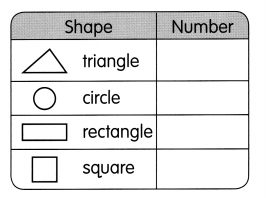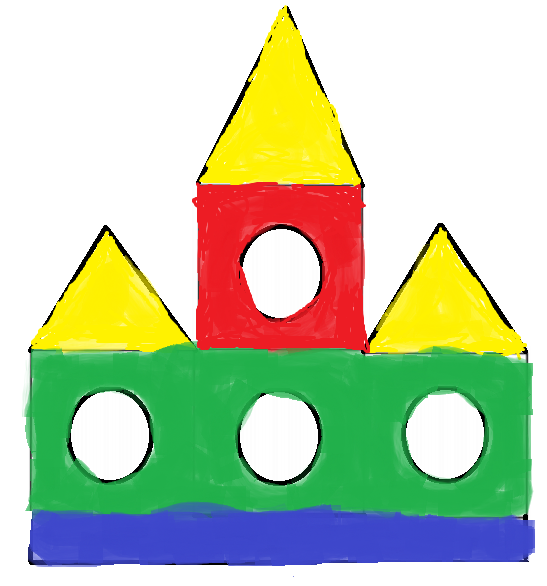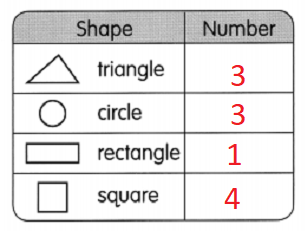# Math in Focus Grade 1 Chapter 5 Practice 4 Answer Key Making Pictures and Models with Shapes

This handy Math in Focus Grade 1 Workbook Answer Key Chapter 5 Practice 4 Making Pictures and Models with Shapes detailed solutions for the textbook questions.

## Math in Focus Grade 1 Chapter 5 Practice 4 Answer Key Making Pictures and Models with Shapes

Find the shapes in the pictures.

Count how many of each shape there are. Write the number.

Question 1.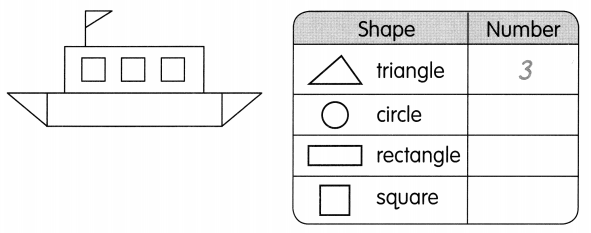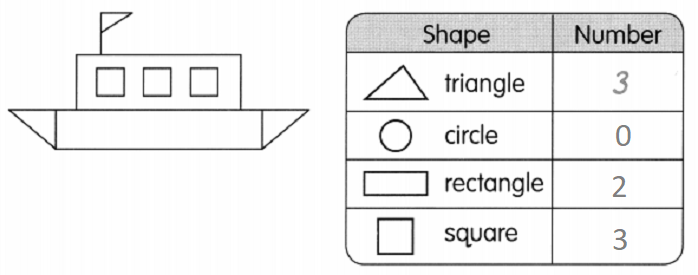Question 2.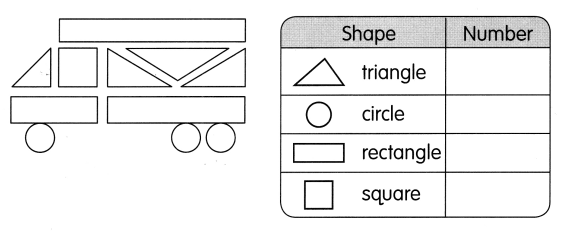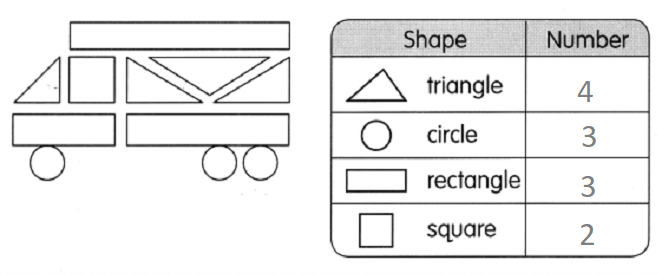Match the pieces to make a shape. Name the shapes. Use the words in the box.

Question 3.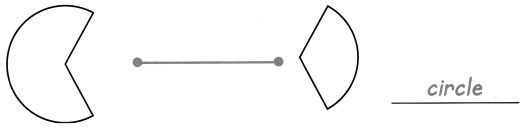Question 4.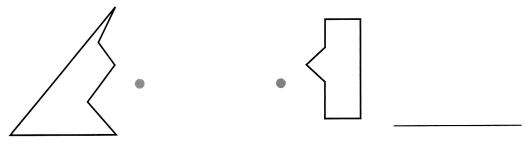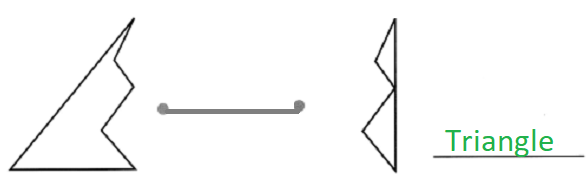Question 5.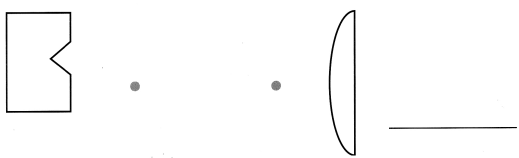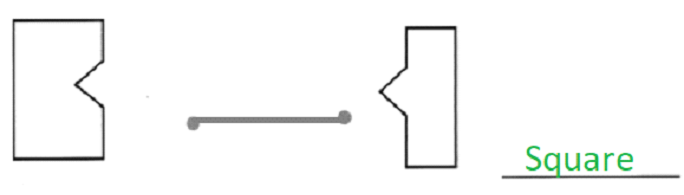Question 6.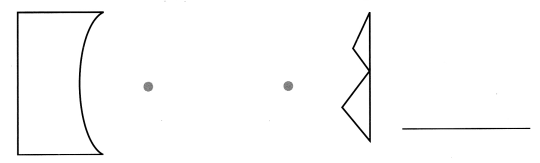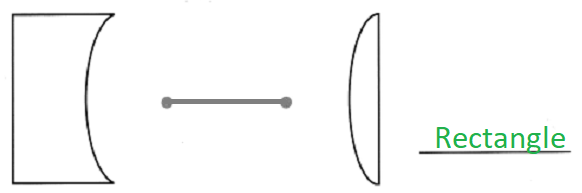Cut out the shapes below and make a picture. Paste the picture here or use your own paper. You do not need to use all the shapes.

Question 7.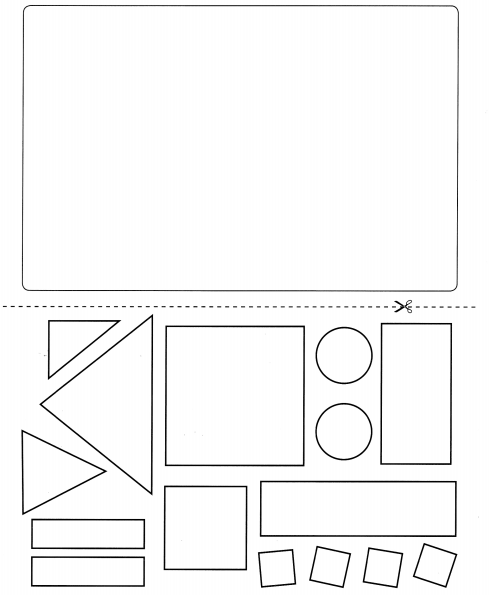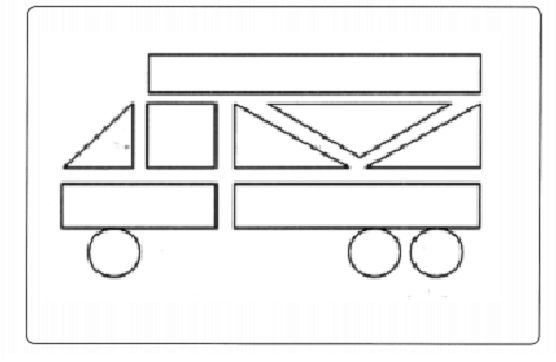Look at the pictures. Then fill in the blanks.

Question 8.
How many triangles can you see?
I can see ___ triangles.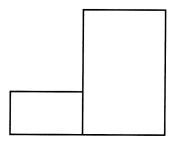Answer: In the above figure there are 0 triangles.

Question 9.
A star can be made of triangles.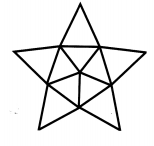This star is made of _____ triangles.
This star is made of 10 triangles.
Draw triangles another way to make up this star.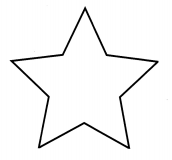This star is made of ___ triangles.